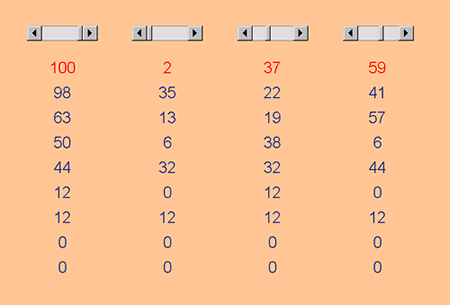# Excel Investigation: Difference Tuples

##### Age 11 to 16Challenge Level

This is based on the activity Differs.

Here's the Excel file I created: Difference Tuples. I usually right-click, "Save Target As", and select where I want the file to be saved.You can see the given start numbers 100, 2, 37, 59 in the first row.

Here are the formulae I used (you can see these in the formula bar when you click on each cell).

ABS calculates the positive difference.

Under 100, =ABS(A2-B2) this compares 100 with 2
Under 2, =ABS(B2-C2) this compares 2 with 37
Under 37, =ABS(C2-D2) this compares 37 with 59
Under 59, =ABS(D2-A2) this compares 59 with 100, right back at the beginning.

Once I had all four formulae in and working correctly I highlighted these four cells (A3 to D3). I then caught hold of the extreme, bottom, right-hand corner of the highlighted area (the normal white cross changes to a small thin black one), and I dragged down as far as I wanted those formulae to be replicated. Excel created new formulae which do the same job as the formulae in the top line, but automatically adjusted the cell references so that they would be correct for the new position.

Isn't it nice when you get answers that quickly? It leaves time to ask more interesting questions.

### For example:

Which set of start numbers gives the longest run before becoming 0 0 0 0?
How is the 4-number problem like a pair of 2-number problems running side by side? How is it different? How is the 8-number problem like a pair of 4-number problems running side by side? How is it different?

Have I missed anything? Oh yes, the increment buttons:
So that I could change the start numbers quickly, and without typing, I put in some scroll bars to control the values in the first row. Look back at October 02 to find out about increment buttons. They're very easy and let you keep your eye on the numbers instead of the keyboard.